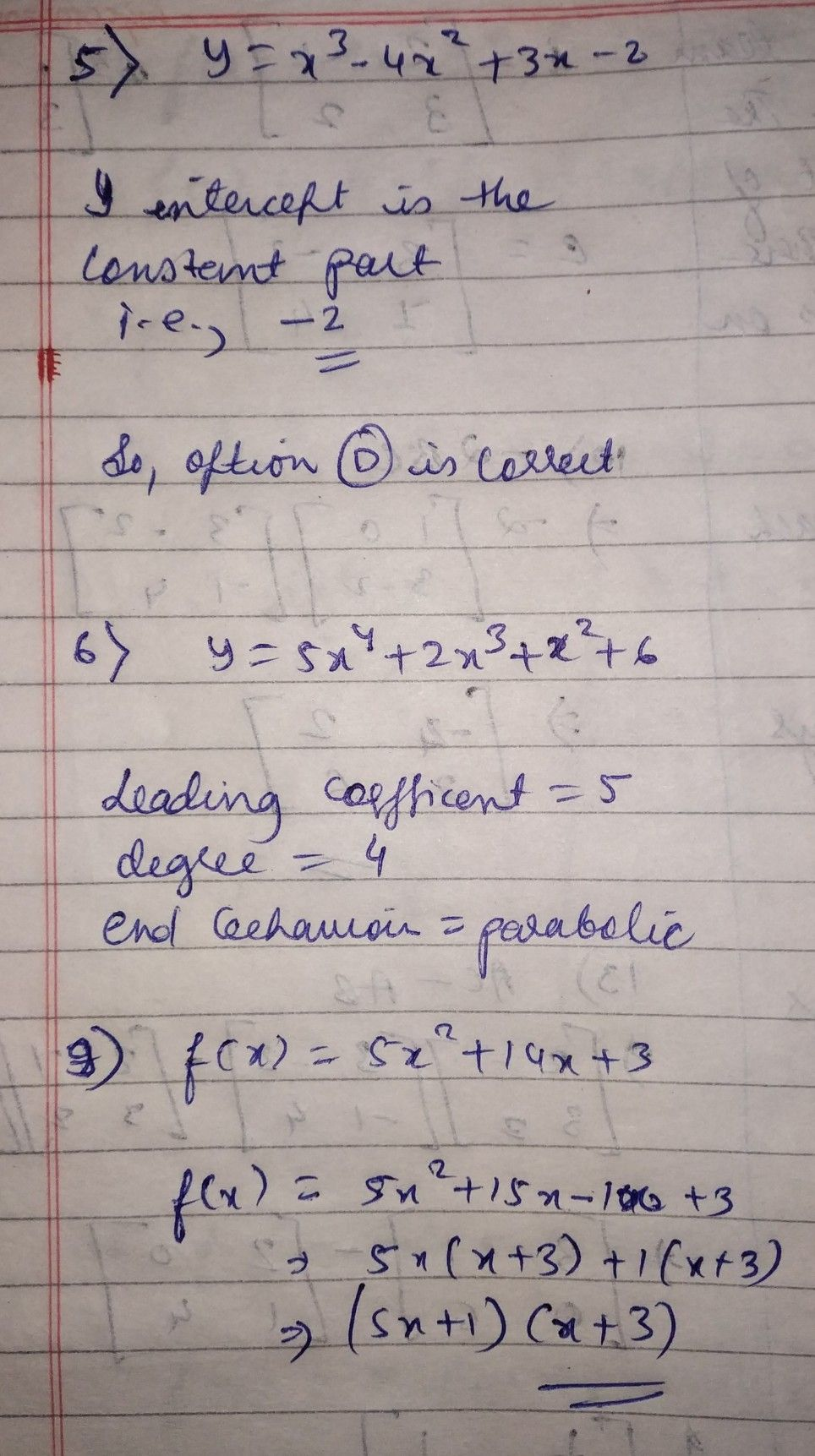Symbol
Problem$D$ $5$ What is the $y-$ intercept of the function $y=x3-4x2+3x-27$ $A4$ $B-4$ $3$ C. $2$ $0.-2$ $6-8$ Identify the Leading coefficient, degree, and end behavior. $y=-5x^{n}4+2x^{n}3+x^{n}2+6$ $910$ Factor the palynomial $f\left(x\right)=5x^{A}2+14x-3$ $\left($ (show your solution at the back)